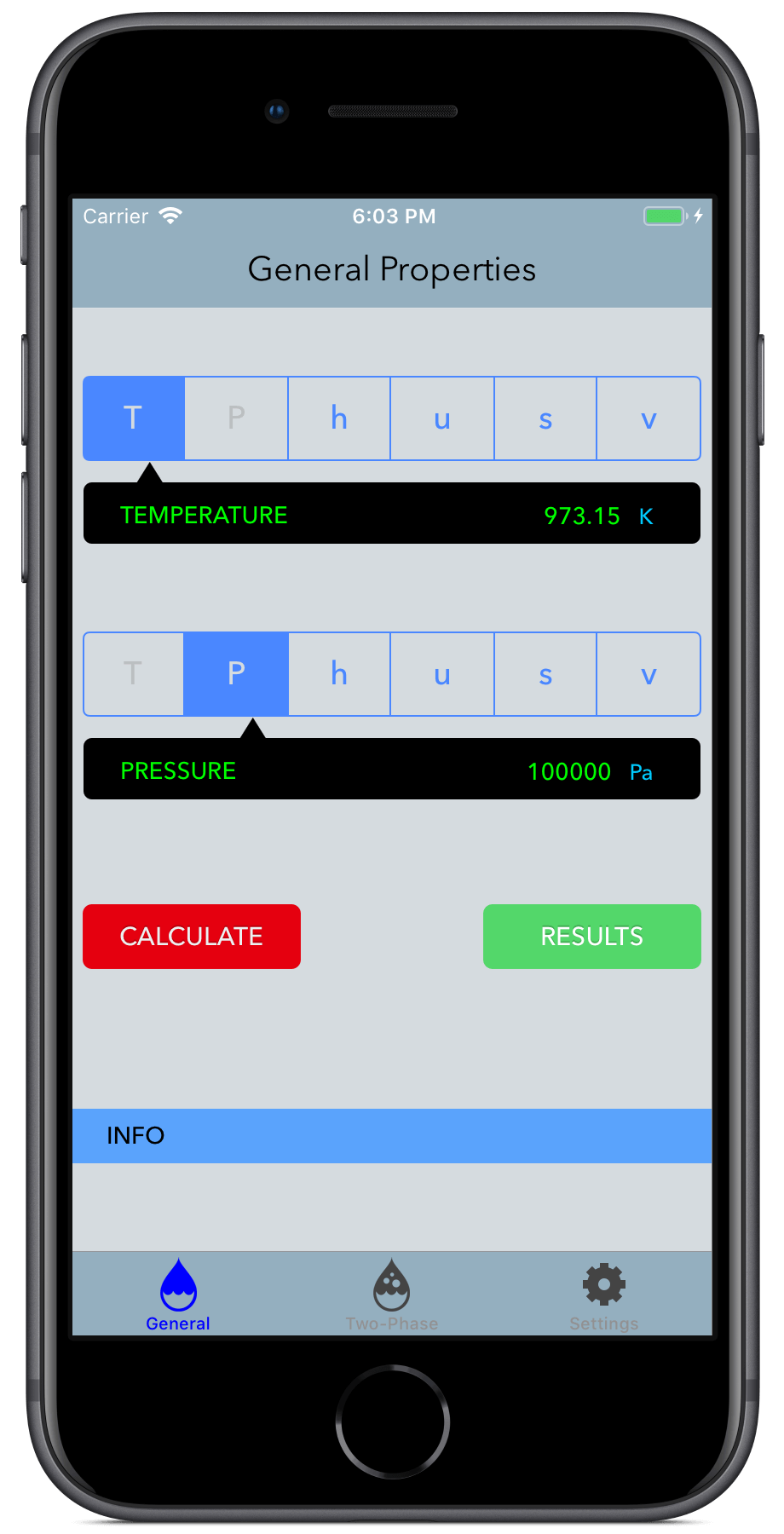Steam IF97
for iPhoneREQUIREMENTS

• Requires iOS 12.1 or later. Compatible with iPhone.

FEATURES

• Implements the mathematical formulation from the IAPWS-IF97 to calculate the properties of steam.
• Calculates 17 Thermodynamic and Transport properties of steam.
• Calculations all over the range of the IAPWS-IF97, or just the two-phase region including liquid and vapor saturation states.
• It allows for 14 combinations of thermodynamic properties to be entered.
• Validates each calculation informing the user of the range of values to be entered in case of an invalid input.
• It manages and converts results and inputs between the SI (metric) and I-P (English) System of Units.
• Results can be sent by email along with a comma-separated value (CSV) file.

Steam IF97 performs calculations of Thermodynamic and Transport properties of steam based on the latest IAPWS-IF97 Formulation and IAPWS releases.

Given a combination of two thermodynamic properties, it calculates 17 Thermodynamic and Transport properties of steam.

The numerical results obtained are suitable for engineering, scientific or industrial use.

MAIN FEATURES

• Performs all the calculations implementing the latest mathematical formulations from the IAPWS (International Association for the Properties of Water and Steam) Industrial formulation (Revision 2007):
• Industrial Formulation 1997 for the Thermodynamic Properties of Water and Steam.
• Properties of steam, water and ice from the Industrial Formulation IAPWS-IF97, the Scientific Formulation IAPWS-95, IAPWS Formulation 2008 and IAPWS Formulation 2006. Properties of dry air are from the NIST Reference equation of Lemmon et al.

• Calculations operate in two modes:
• General Properties: Input and Output values are all over the range of the IAPWS-IF97 Formulation.
• Saturation Properties: Input and Output values are on the range of the two-phase region (region 4) of the IAPWS-IF97 Formulation, including saturation values of liquid and vapor.

• Allows for 14 different combinations of thermodynamic properties to be entered as input parameters:
• Temperature / Pressure
• Temperature / Specific Enthalpy
• Temperature / Specific Internal Energy
• Temperature / Specific Entropy
• Temperature / Specific Volume
• Temperature / Vapor Fraction
• Pressure / Specific Enthalpy
• Pressure / Specific Internal Energy
• Pressure / Specific Entropy
• Pressure / Specific Volume
• Pressure / Vapor Fraction
• Specific Enthalpy / Specific Entropy
• Specific Enthalpy / Specific Volume
• Specific Internal Energy / Specific Volume

• Supports input parameters and calculation results in both the SI (metric) and the I-P (English) system of units.

• For each combination of input thermodynamic properties, it calculates and provides the user with information about the appropriate input values in the valid range of computations.

• Results can be sent by email in an HTML file along with a comma-separated value (CSV) file.

SYSTEM OF UNITS

Thermodynamic variables in the SI (metric) system of units can be entered and/or the calculation results can be displayed in the following units:

• Pressure: Pa, kPa, bar, mmHg
• Temperature: °C, K
• Specific Enthalpy: kJ/kg, J/kg, kcal/kg
• Specific Entropy: kJ/(kg·K), J/(kg·K), kcal/(kg·K)
• Specific Internal Energy: kJ/kg, J/kg, kcal/kg
• Specific Volume: m³/kg, cm³/g, l/kg
• Density: kg/m³, g/cm³, kg/l
• Speed of Sound: m/s, km/h
• Dynamic Viscosity: Pa·s, kg/(m·s), P
• Kinematic Viscosity: m²/s, St
• Thermal Conductivity: W/(m·K), kcal/(m·s·K)
• Thermal Diffusivity: m²/s, m²/h

Thermodynamic variables in the I-P (English) system of units can be entered and/or the calculation results can be displayed in the following units:

• Pressure: psi, inHg, inH2O, atm
• Temperature: °F, °R
• Specific Enthalpy: Btu/lbm, ft lbf/lbm
• Specific Entropy: Btu/(lbm·°R), ft lbf/(lbm·°R)
• Specific Internal Energy: Btu/lbm, ft lbf/lbm
• Specific Volume: ft³/lbm, in³/lbm, gal(US)/lbm
• Density: lbm/ft³, lbm/in³, lbm/gal(US)
• Speed of Sound: ft/s, mile/h
• Dynamic Viscosity: lbm/(ft·s), lbf·s/ft²
• Kinematic Viscosity: ft²/s, ft²/h
• Thermal Conductivity: Btu/(ft·h·°R), W/(in·°F)
• Thermal Diffusivity: ft²/s, ft²/h

Output Variables

• Vapor Fraction
• Temperature
• Pressure
• Specific Volume
• Density
• Specific Enthalpy
• Specific Entropy
• Specific Internal Energy
• Isobaric Heat Capacity
• Isobaric Cubic Expansion Coefficient
• Isentropic Exponent
• Isothermal Compressibility
• Thermal Conductivity
• Thermal Diffusivity
• Dynamic Viscosity
• Kinematic Viscosity
• Prandtl Number
• IAPWS-IF97 Region

For the calculation of Saturation Properties, it calculates all the properties shown above for results in region 4 of the IAPWS-IF97 Formulation, and the same properties for the Saturated Liquid State and Saturated Vapor States.

Range of Input/Output Variables (SI)

Property

Valid range

Units

Temperature

0 ≤ T ≤ 2000

°C

Pressure

610 ≤ P ≤ 100.0E6

Pa

Specific Enthalpy

-41.5878 ≤ h ≤ 2810.0E3

J/kg

Specific Internal Energy

-282.7252 ≤ u ≤ 6327.862E3

J/kg

Specific Entropy

-8.5823 ≤ s ≤ 13905.8727

J/(kg·K)

Specific Volume

1.00007E-4 ≤ v ≤ 1719.8658

m³/kg

Vapor Fraction

0 ≤ x ≤ 1.0

kg/kg

Range of Input/Output Variables (I-P)

Property

Valid range

Units

Temperature

32.0 ≤ T ≤ 3632.0

°F

Pressure

0.088473 ≤ P ≤ 1450.4

psi

Specific Enthalpy

-0.0178795 ≤ h ≤ 3171.5306

Btu/lbm

Specific Internal Energy

-0.12155 ≤ u ≤ 2720.491

Btu/lbm

Specific Entropy

-0.0020498 ≤ h ≤ 3.32136

Btu/(lbm·°R)

Specific Volume

1.601958E-2 ≤ v ≤ 27549.6

ft³/lbm

Vapor Fraction

0 ≤ x ≤ 1.0

lb/lb

Notice

This website or its third-party tools use cookies, which are necessary to its functioning and required to achieve the purposes illustrated in the cookie policy. By closing this banner, scrolling this page, clicking a link or continuing to browse otherwise, you agree to the use of cookies. Please refer to our Cookie Policy, revised Privacy Policy and Terms and Conditions.